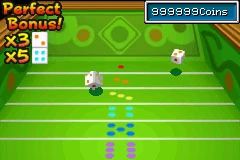## FANDOM

1,307 PagesLucky Dice is located in Tolbi and Contigo. Lucky Dice is a gambling minigame in Golden Sun and Golden Sun: The Lost Age. It is a very simple game that can be found later on in both game carts, and prizes are strictly centered on coins. In The Lost Age, a second Dice-based game called Super Lucky Dice is added.

## RulesEdit

Gameplay takes place on a green square table reminiscent of Craps and Roulette tables seen in modern-day casino games. The table is divided into six equally spaced regions, each representing a value between one and six like the values on a die. The object of the game is to throw a pair of dice into the table and hope for matching numbers based on what the dice show and where the dice land.

### BettingEdit

Betting is a very simple process; the moment you throw dice, the game automatically bets your money for you. The value of the bet is determined by the experience level of your party; the bet is equal to the lowest level of any of your party members, multiplied by ten. Therefore, if Ivan were at level 28 while Isaac were at level 26, the bet would calculate to 260 coins. Likewise, a level 54 Felix out of a party of level 52 Adepts would cause the bet to be 520 coins.

### OutcomesEdit

At any rate, once you throw a pair of dice and the bet value is deducted from your total coin account, the game takes over. When the two thrown dice land, not two but four values are returned: the two values of the rolled dice themselves and the values of the regions on the board where the dice physically land. For example, if the two dice themselves return values of 4 and 6, and one of these dice lands on the 2 region of the board while the other lands on the 4 region, the values returned are one 2, two 4s, and one 6. The outcome of this would be a Pair.

Now for the outcomes themselves: if a Pair of matching values are returned from the four values returned by the dice roll, then you Break Even; that is, you regain the value of your bet. You have neither lost any coins nor won any. If you get Two Pair, you gain twice as much money as what you’ve bet, so that if you bet 290 coins you will end up 290 coins richer. If three of the four values match, it is a Triple, and you gain three times as much money as what you’ve bet (you end up with 580 more coins than you did before starting the game). The most desirable outcome is when all four values match, achieved when both dice match and both land in the same region, a region that matches the values of the Dice. Four of a kind in this case is called a Perfect, and you gain five times as much money as what you’ve bet (you end up with 1160 coins if your bet was 290).

### Perfect BonusEdit

An additional element to increase the potential for winnings is called the Perfect Bonus. At each game played, two of the six dice values chosen at random will have multipliers next to them shown on the upper left of the screen. This means that if you roll four of a kind of the value next to the multiplier, your total winnings will be multiplied accordingly. This is the equivalent to the Jackpot value. In the example pictured, the four-of-a-kind rolled is based on a value of two, which currently has a Perfect Bonus multiplier of 3, so the payout is 15 times the value of the original bet instead of five times. The highest payout for Lucky Dice possible, based on having a level 99 party and rolling a four-of-a-kind based on a value with a multiplier of five, comes to 24,750 coins.

## StrategyEdit

A strategic element manifests itself in the form of being able to control how high you physically throw the dice. You are able to position your hands to determine the height and distance the dice are thrown across the table, which can influence where the dice end up.

The expected value (or expected gain) for a game of Lucky Dice, assuming that all throws result in perfectly random results, is .018 * your initial bet (calculated as (-1 * probability of no match) + (0 * probability of one pair) + (1 * probability of two pair) + (2 * probability of three of a kind) + (9 * probability of a perfect); the 9 comes from the average gain from a perfect, which is 4*2/3+14*1/6+24*1/6, or 9), which shows that, statistically, the game should tend to favor you in the long run.

However, an analysis of over 400,000 dice throws shows that some throwing positions result in better gains than others. The optimal way to throw the dice appears to be a little below the maximum height:

For reference, the initial throwing position is at 96px, and the lowest and highest positions are 32px and 160px respectively. Tapping the up and down keys for 1 frame increase / decrease the position by 4px.

Another strategy is to adjust the throwing distance to attempt to increase the chance that the dice will land in a board area with a Perfect Bonus currently attached to it. See the following table for the optimal throwing position to most likely land the dice in a specific area:

Community content is available under CC-BY-SA unless otherwise noted.